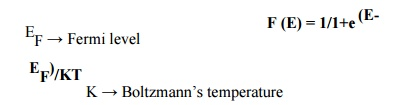Home | | Engineering Physics II | Important Questions and Answers: Conducting Materials

# Important Questions and Answers: Conducting Materials

Physics - Conducting Materials - Important Questions and Answers: Conducting Materials

CONDUCTING MATERIALS

1. What are the merits of classical free electron theory?

(i) It is used to verify Ohm’slaw.

(ii) It is used to explain electrical and thermal conductivities of metals.

(iii)It is used to derive Wiedemann –Franz law.

(iv)It is used to explain the optical properties of metal.

2. What are the drawbacks of classical free electron theory?

(i)   Classical theory states that all free electrons will absorb the supplied energy; on the contrary, quantum theory states that only a few electrons will absorb the supplied energy.

(ii)   Electrical conductivity of semiconductors and insulators (non –metal) could not be explained by this theory.

(iii)   The phenomena such as photo –electric effect, Compton effect and black body radiation could not be explained on the basis of this theory because these phenomena are based on quantum theory.

3. Define mean free path.

The average distance traveled by a free electron between any two successive collisions in the presence of an applied field is known as mean free path. It is the product of drift velocity of the electron (vd) and collision time (τ)

λ= vd ×τc

4. Define relaxation time of an electron.

The average time taken by a free electron to reach its equilibrium position from its disturbed position due to application of an external electrical field is called relaxation time.

5. Define drift velocity of electron. How is it different from the thermal velocity of an electron?

The average velocity acquired by a free electron in a particular direction after a steady state is reached on the application of an electrical field is called drift velocity. It is denoted as vd..

The thermal velocity is random in nature and its value is very high (105 m/s), but the drift velocity is unidirectional and its value is very small (50 cm/s).

6. Define mobility of electrons.

The magnitude of the drift velocity per unit electric field is defined as the mobility of

electrons (μ)

i.e., μ= vd/E

Where vd→ drift velocity of electrons E→ lectricalE field.

7. Define electrical conductivity.

It is the amount of electrical charge (q) conducted per unit time (t) across unit area (A) of the solid per unit applied electrical field (E).

σ= q/tAE

8. State Wiedemann –Franz law.

It states that the ratio of thermal conductivity (K) to electrical conductivity (σ)ofa metal is directly proportional to absolute temperature (T) and this ratio is constant for all metals at a given temperature.

K/ σ∞T i.e., K/ σ= LT

Where L is a constant and it is known as Lorentz number.

9. What is Lorentz number?

The ratio  between  thermal  conductivity  (K)  of  a  metal  to  the  product  of

electrical conductivity (σ)ofa metal and absolute temperature (T) of the metal is a constant. It is called Lorentz number and it is given by

L = K/σT

10. Define Fermi distribution function.

The probability F (E) of an electron occupying a given energy level at temperature T is known as Fermi distribution function. It is given byE    →Energy of the level whose occupancy is being considered.

11. Define Fermi level and Fermi energy with its importance.

Fermi level is the energy level at finite temperature above 0K in which the probability of the electron occupation is ½ and it is also the level of maximum energy of the filled states at 0K

Fermi energy is the energy of the state at which the probability of the electron occupation is ½ at any temperature above 0K.It is also the maximum energy of filled states at 0K.

Importance Fermi level and Fermi energy determine the probability of an electron occupying a given energy level at a given temperature.

12. Define density of states. What is its use?

It is defined as the number of available electron states per unit volume in an energy  interval E and E+dE. It is denoted by Z (E). It is  used to determine Fermi energy at any temperature.

13. What are difference between Drift velocity and thermal velocity of an electron?Drift Velocity

Drift velocity is the average velocity acquired by the free electron, in the presence of electric field.

The electrons moving with drift velocity moves in the direction opposite to that of the field direction.

The velocity is very less, say in the order of 0.5 m/s.

Thermal Velocity

Thermal velocity is the velocity of an electron without any external field.

The direction of the electrons moving with thermal velocity is random.

The velocity is very high, say in the order of 10 5 m/s.

14. Distinguish between electrical conductivity and thermal conductivity.S.No Electrical Conductivity

1. The co-efficient of electrical conductivity is defined as the quantity of electricity flowing per unit area per unit time maintained at unit potential gradient.

2. Electrical conductivity is purely due to number of free electrons.

3. Conduction of electricity takes place from higher potential end to the lower potential end.

4. Unit: ohm -1 m-1

Thermal Conductivity

The Co-efficient of thermal conductivity is defined as the quantity of heat conducted per unit area per unit time maintained at unit temperature gradient.

Thermal conductivity is due to both free electrons and phonons.

Conduction of heat takes place from hot end to cold end

Unit: Wm -1 K-1

Study Material, Lecturing Notes, Assignment, Reference, Wiki description explanation, brief detail
Physics : Conducting Materials : Important Questions and Answers: Conducting Materials |